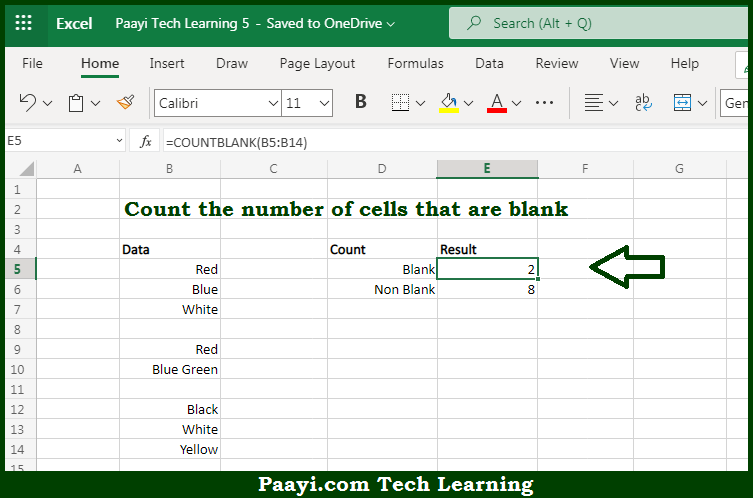# Learn How to COUNT Cells that are Blank in Microsoft Excel

Written by | 0 Comments | 551 Views

In this article, you will learn how to COUNT various things in Microsoft Excel using a single or combination of functions and its purpose in Microsoft Excel. You will also get to know how to COUNT cells that are blank and see the generic formula.

Count Cells that are Blank in Microsoft Excel

The main purpose of this formula is to count cells that are blank. Here we will learn how to count cells that contain not any data in Microsoft Excel.  That implies, with the help of a formula based on the  COUNTBLANK function you can able to count cells that do not contain any data or which are blank. So, with the help of this formula, you can able to count cells that are blank.

General Formula to COUNT Cells that are Blank

=COUNTBLANK(range)

The Explanation for the COUNT Cells that are BlankSo we know that with the help of the given formula above you can able to count cells that are blank. Here we will learn how to count cells that contain not any data in Microsoft Excel. As we know that, The COUNTBLANK function is used to count the number of cells in the range that don't contain any value and return this in the form of a number as the result. It should be noted that the cells that contain text, numbers, dates, errors, etc. are not counted and the COUNTBLANK function is fully automatic. You just need to pass in a range, and the function will return a result. So, with the help of the COUNT formula, you can able to count cells that are blank.